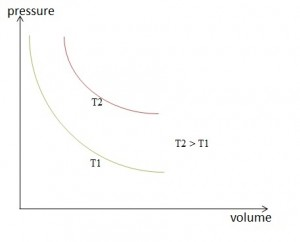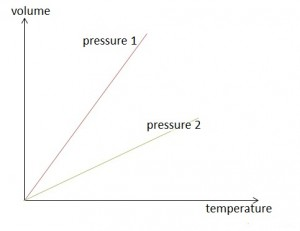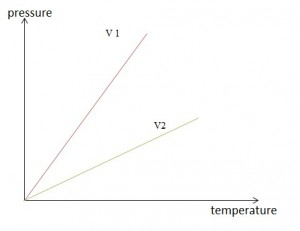Blood is responsible for preventing the body from cooling down, while sweat prevents it from overheating. But how exactly does this work? Basic knowledge of thermodynamics is necessary in order to understand heat transfer, the functions of substance mixtures, and the relevance of different pressures inside the human body. What is a gas? What are the properties of different substances, and how can they be described? The following article deals with these basic principles of physics.
Are you more of a visual learner? Check out our online video lectures and start your physics course now for free!Thermal State Variables – Pressure, Volume, Temperature

Pressure and Pressure Propagation in Liquids and Gases

 p pressure [Pa], [N/m2] F force acting on a surface[N] A area of the surface, cross-section [m2]

Liquids have no definite shape due to the mutual relocatability of molecules or atoms. They assume the shape of the available vessel. As a whole, the surface of liquids forms a horizontal plane. In gases, there are no binding forces between the individual molecules. The molecules are – except at absolute zero – constantly in motion. If they collide or bounce against surrounding walls, they are repelled elastically. Thus, they move on irregular zigzag paths.

A gas molecule undergoes about 10 quadrillion collisions per second.

In a fully sealed vessel, force can be exerted on a fluid by means of a movable stamp. Unlike solids, where a force can be exerted on one point, the particles of a fluid can avoid contact due to their movability. With a stamp, the force can act evenly through the entire surface of the stamp. The particles adjacent to the surface of the stamp experience an immediate pressure which they pass on in all directions due to their mobility. Therefore, the pressure is the same at any point within a fluid.

Pressure is defined as the ratio of a force applied perpendicular to a surface and the area of this surface: p = F / A.

Pressure and Volume of a Gas

The volume and pressure of a gas have an inverse relationship where if one increases the other decreases. At constant temperature, the pressure of a gas is proportional to the number of molecules present in the respective area. A measure for the number of molecules is the mass of the gas.

Boyle-Mariotte law:
At constant temperature and in a closed container, the product of pressure and volume of a given amount of gas is constant.
• PV=constant
• p1v1-p2v2 is Boyle-Mariotte lawSince a change in pressure affects the volume of a gas quantity, the density of gases must be pressure-dependent as well. At constant temperature, the density of a gas is proportional to the pressure. Densities behave the same as pressures.

Real and Ideal Gases

Real gases have a volume thus they occupy space, they collide with each other with motion being slowed or increased and their particles are in constant interaction with each other.

In order to calculate gases easier, the following simplifications have been defined:

• Particles are elastic bodies of negligible small volumes.
• Elastic reflections at the vessel walls occur.
• Particles are evenly distributed (homogeneity).
• The velocities of the particles are evenly distributed (isotropy).

Ideal gases have no definite volume, they have no mass, and their particles’ collision is elastic. When their particles collide there is no energy involved and their pressure is higher compared to that of ideal gases.

Equation of State for Ideal Gases

 V Volume [l] m Mass [kg] M Molar mass n Amount of substance [mol] R Universal gas constant [J/(K*mol)] T Temperature [K], [°C]

For description of ideal gases, a general equation of state (the ideal gas law) is used. It is defined as the simultaneous change of volume, pressure and temperature.

p * V = m * R * T

In this equation, absolute pressure must be used. The ideal gas law does not apply in conditions of overpressure. Furthermore, it only applies to ideal gases and has only limited validity in regard to real gases and none in regard to vapors. From the ideal gas law, a body’s standard volume can be calculated. Standard volume refers to the volume at standard atmospheric pressure p= 1013 mbar and at a temperature of T = 0 °C. At these standard conditions (STP), 1 mole of an ideal gas occupies a volume of V= 22.4 l.

If the constant term p * V / T is proportional to the mass of the closed system, the associated proportionality factor is referred to as gas constant. The gas constant’s numerical value depends on the type of gas.

The number of similar particles contained in a system is described as amount of substance. A mole is the amount of a substance that contains as many particles as there are atoms in 12 grams of a carbon isotope C12. So in all substances, an amount of 1 mole contains 6.0221367 * 1023 particles. At standard conditions, 1 mole of any gas has the same volume (see standard volume).

The product of universal gas constant with molar mass is called the molar gas constant. It is a universal constant and thus, non-specific to substances.

p * V = n * M * R * T

Equation of state in relation to amount of substance:

p * V = n * R * T

Thus, the state of a gas is determined by three state variables: pressure, volume and temperature. Changes in two or all state variables are referred to as change of state.

There is a clearly defined value of internal energy for each state of a system. To understand these changes, we remember the first law of thermodynamics (see Thermodynamics I):

The supply of heat and mechanical work increases the internal energy of a closed system. The following applies:

ΔU = Q + W

In ideal gases, mechanical work causes a volume change. The supplied thermal energy leads to an increase of the internal energy and an increase in volume.

 Isothermal change of state Change of state of an ideal gas is isothermal when temperature remains constant. p * V = constantIsobaric change of state Change of state of an ideal gas is isobaric when pressure remains constant. V / T = constantIsochoric change of state Change of state of an ideal gas is isochoric when volume remains constant. ρ / T = constantK Adiabatic exponent ΔS Change of entropy (S = 0 in adiabatic change) Cp Isobaric heat capacity [J/(kg*K)] CV Isochoric heat capacity [J/(kg*K)]

Adiabatic change of an ideal gas takes place without heat exchange with the environment. Complete heat insulation is required for this change of state. Adiabatic changes of state are also referred to as isentropic changes of state. The corresponding equation for an isentropic change of state is:

p * VK = const; K = CP / CV

The work performed in an isentropic relaxation is, when the amount of gas is given, only dependent on the change in temperature.

Gas Mixtures

 ρA Partial pressure of a gas [bar] A Concentration of a gas in a solvent [mole/l] K Constant

Mixtures of at least two different chemical elements that result in a gas are called gas mixtures. They have a homogeneous mixing ratio.

The solubility of a gas in a liquid is described by Henry’s law. The gas concentration in the liquid is proportional to the partial pressure of the gas above the liquid.

A = K · pA

States of Aggregation

Substances can assume the following states of aggregation:

• Solid
• Liquid
• GaseousEach of these three states of matter implies specific properties for a substance. Solid substances have a fixed shape and volume. Their particles are held together by cohesive forces. Liquid substances have no fixed shape and take up the shape of the container holding them. However, they have a fixed volume and cohesive forces are acting between their particles. Gaseous substances neither possess a definite shape, nor a certain volume. Their particles exert only negligible cohesive forces. These are the primary states of matter as they all occupy space and have weight despite the differences in terms of cohesive bonds amongst the particles.

To reach a higher state of aggregation (solid < liquid < gaseous), energy must be added. When a change into a lower state of aggregation takes place, energy is released.

Melting and Solidifying

The transition from a solid state into a liquid state is called melting. The reverse change is called solidification.

Melting of a body occurs at its melting temperature. Melting and solidification temperature are the same for one substance. During melting, the melting temperature remains constant despite the adding of heat. The added heat is used to transfer the substance from solid to liquid. The same applies to solidification: The temperature remains constant despite the release of heat. Only after the entire substance has solidified, further heat dissipation results in the cooling of the solid material. These two processes shows how heat energy affects the cohesive bonds in solids to make them weaker thus it turns into liquid.

Vaporization and Condensation

Processes in which a substance changes from a liquid to a gaseous state are called vaporization. Reverse processes are called condensation.

There are two types of vaporization: Boiling implies the evaporation of a liquid where vapor bubbles form inside the liquid phase. The temperature of the liquid remains constant during boiling despite further heat supply. The boiling temperature is strongly dependent on the pressure of the liquid. Normal boiling temperature refers to the boiling temperature at atmospheric pressure of 1013 mbar. The other type of vaporization, where a liquid vaporizes below its boiling temperature, is called evaporation. It occurs only on the liquid surface and may occur at every temperature above zero. During evaporation, a liquid draws the required heat from its own energy content and from the environment.

Example: When a person is sweating, the sweat evaporates from the surface of the skin. Energy in the form of heat is released. The body gives off excess heat to prevent overheating.

Through evaporation and condensation of water, “pure” water can be produced. This type of liquid treatment is called distillation. It is a commonly recommended way of purifying water for drinking at domestic and commercial levels.

Sublimation

Many substances change directly from the solid to the gaseous state and vice versa, without any formation of liquid. This can be observed in the volatilization of naphthalene or the formation of ice crystals in the air. This phase transition from solid to gas and vice versa is called sublimation. The liquid state is skipped.

Vapor Pressure

In the case of ideal gases, pressure and volume are inversely proportional to each other. In the case of real gases, this inverse proportionality is only accurate by approximation. When vapors change in volume, the pressure hardly changes at all. This difference between gases and vapors is based on the difference between the temperature and the pressure-dependent boiling point.

Vapor pressure (p) does not depend on the volume of the vapor. It only depends on the temperature.

The vapor is saturated when the saturation vapor pressure is reached, i.e., when it is at equilibrium with the liquid’s pressure.

Gases are highly unsaturated vapors. Their temperature is far above the boiling point that corresponds to their pressure.

Water Vapor in the Air

Since water constantly evaporates from the surface of all natural bodies of water, the air always contains water vapor, which is mostly unsaturated. To become saturated, it must be cooled to a specific temperature which is called dew point. In case of cooling below the dew point, so much water vapor is condensed that only the water vapor content corresponding to this temperature remains. The condensed water vapor appears in the form of fog, rain, snow or frost.

At each temperature, only a certain amount of water vapor can be contained in a certain volume of air. The water vapor content in the atmospheric air varies regarding time and location and is referred to as humidity. The maximum humidity is defined as the maximum amount of water vapor that can be contained in one cubic meter of air at a certain temperature.

F (maximum) = Mass of water vapor in the air / Volume of humid air

Critical Temperature

The critical temperature of a gas is the temperature at which liquefaction is no longer possible, regardless of how high the pressure is. The corresponding isothermal curve shows an inflection point with a horizontal tangent at the critical pressure. Only below the critical temperature gases can be liquefied by applying pressure.

Real gases slightly cool down during a reduced relaxation.

Triple pointThe three mentioned states of aggregation can reach a joint thermodynamic equilibrium. If this equilibrium, defined by pressure and temperature, is present, this is called triple point.

Review Questions

Solutions can be found below the sources.

1. On one stamp (surface 100 cm2) of a hydraulic press, a force of 15 N is applied, where during the compression of a sample, a distance of 8 cm is covered. The force applied on the other stamp (surface 400 cm2) and the distance traveled by this stamp are given by:

1. 7.5 N, 16 cm
2. 30 N, 4 cm
3. 30 N, 2 cm
4. 60 N, 4 cm
5. 60 N, 2 cm

2. The plunger of a 50 ml syringe is fully depressed and the syringe is hermetically sealed at the top (see illustration). Which of the following variables are needed to calculate the force that is required to pull out the plunger from the syringe? (friction is negligible)(1) Interior cross-section of the syringe

(2) Volume of the syringe

(3) Air pressure

(4) Angle β according to drawing

1. Only 1 and 2 are correct.
2. Only 1 and 3 are correct.
3. Only 1, 2, and 3 are correct.
4. Only 2, 3, and 4 are correct.
5. All 4 are correct.

3. Which statement is correct for a gas in a closed vessel? If a gas molecule collides with the wall, it exerts a force impact on the wall. The sum of all force impacts divided by the elapsed time and the vessel wall surface results in…

1. …the force the molecules exert on the wall.
2. …the total energy of the system.
3. …the total momentum of the system.
4. …the kinetic energy of the molecules in the vessel.
5. …the gas pressure.(Votes: 2, average: 5.00)Loading...